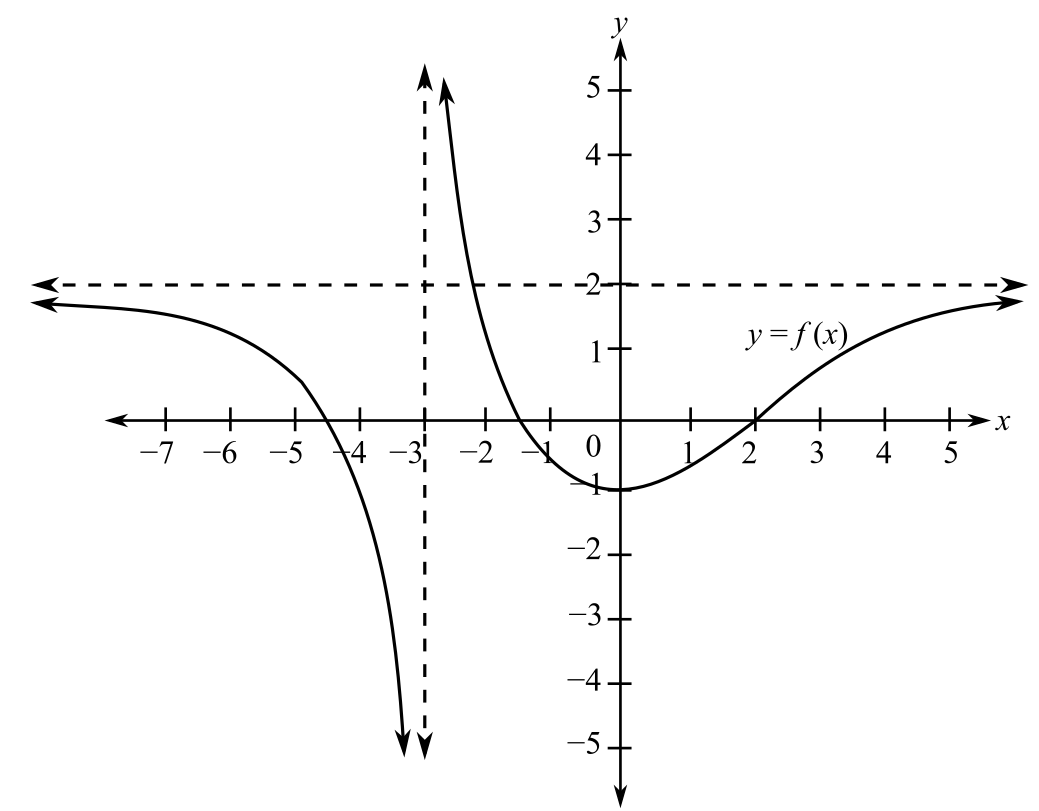Chapter 10, Problem 10T### Mathematical Applications for the ...

11th Edition
Ronald J. Harshbarger + 1 other
ISBN: 9781305108042

#### Solutions

Chapter
Section### Mathematical Applications for the ...

11th Edition
Ronald J. Harshbarger + 1 other
ISBN: 9781305108042
Textbook Problem

# Use the following figure to complete parts (a)-(c).(a) lim x → − ∞ f ( x ) = ? (b) What is the vertical asymptote?(c) Find the horizontal asymptote.(a)

To determine

The value of limxf(x) for the provided graph of a function.Explanation

Given Information:

The provided graph of the function is

.

Explanation:

Consider the provided graph,

Limit of the function limxf(x) is the value of the function when x tends to

(b)

To determine

The vertical asymptote for the provided graph of the function.(c)

To determine

The horizontal asymptote for the provided graph of a function.### Still sussing out bartleby?

Check out a sample textbook solution.

See a sample solution

#### The Solution to Your Study Problems

Bartleby provides explanations to thousands of textbook problems written by our experts, many with advanced degrees!

Get Started